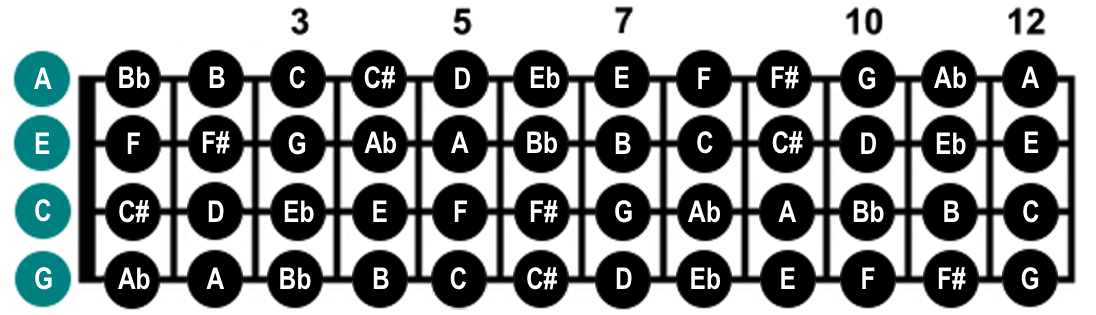Scales

All Scales=   Notes in scale.=   First position scale.=   Open string note not used in scale.

The first position scales use closed string notes, but open string notes can be substituted, although it is advisable to learn the scales using closed string notes.The chart below shows the relationship between the major scale (DO-RE-MI-FA-SO-LA-TI-DO), and chromatic scale, which divides the octaves into twelve half-steps. By using a number for each tone of the major scale, (DO=1, RE=2, MI=3, etc.) a universal key of numbers is created, with which musical relationships learned in one key can be applied to all keys.

For example, the C major scale (C-D-E-F-G-A-B-C) and the A major scale (A-B-C#-D-E-F#-G#-A) can be written as 1-2-3-4-5-6-7-1. Non-major tones can also be written as numbers (Eb in the key of A would be b5).

Tones that are second octave extentions are listed in parentheses. Equivalent tones in the first octave can be found by subtracting seven (since 8=1, then 9=2, #11=#4, etc.).